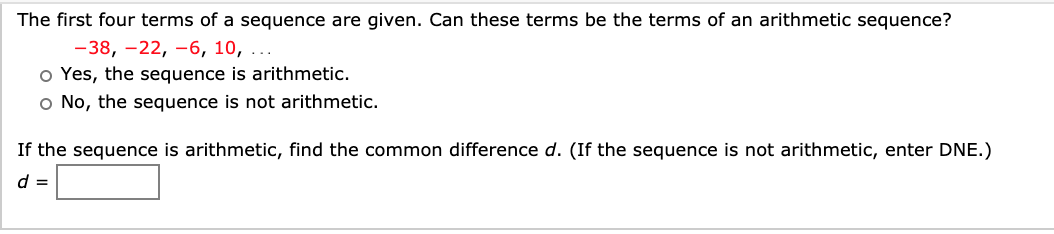# The first four terms of a sequence are given. Can these terms be the terms of an arithmetic sequence? -38, –22, –6, 10, .. o Yes, the sequence is arithmetic. o No, the sequence is not arithmetic. If the sequence is arithmetic, find the common difference d. (If the sequence is not arithmetic, enter DNE.) d =

Questionhelp_outlineImage TranscriptioncloseThe first four terms of a sequence are given. Can these terms be the terms of an arithmetic sequence? -38, –22, –6, 10, .. o Yes, the sequence is arithmetic. o No, the sequence is not arithmetic. If the sequence is arithmetic, find the common difference d. (If the sequence is not arithmetic, enter DNE.) d = fullscreen

### Want to see this answer and more?

Experts are waiting 24/7 to provide step-by-step solutions in as fast as 30 minutes!*

*Response times may vary by subject and question complexity. Median response time is 34 minutes for paid subscribers and may be longer for promotional offers.
Tagged in
MathCalculus

### Other